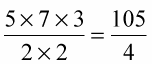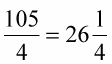##### Basic Math & Pre-Algebra For Dummies Book + Workbook Bundle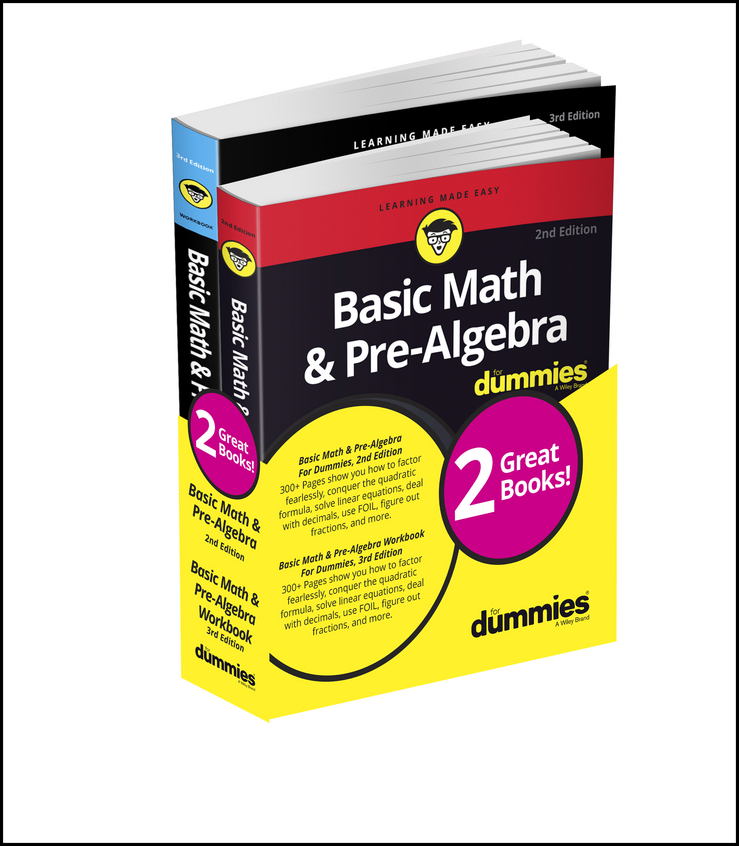Multiplying mixed numbers is quite similar to multiplying simple fractions. Sometimes, you may need to multiply three or more mixed numbers together, for example, when applying one discount after another to an original list price.

To multiply mixed numbers, first change them to improper fractions. Next, simplify, or reduce, the fractions by removing common factors. Then multiply the numerators, and multiply the denominators. Simplify, or reduce the answer if possible. Finally, if the answer is an improper fraction, you can change it back to a mixed number.

Here's an example of a multiplication problem with three mixed numbers: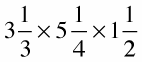1. Change all mixed numbers to improper fractions.

In this example, you have to convert three mixed numbers to improper fractions: 3 1/3, 5 1/4, and 1 1/2. Follow this formula to convert each mixed number into an improper fraction: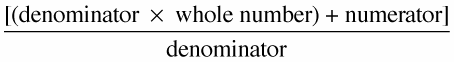In a fraction, the Numerator is always North of (above) the fraction line. The Denominator is always Down below the fraction line.

You convert the mixed numbers to improper fractions as follows: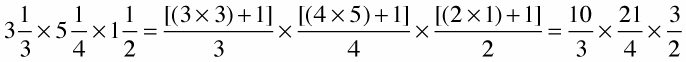2. Reduce the fractions.

To do this, break down both the numerator and denominator of each fraction into their prime factors (shown below in parentheses):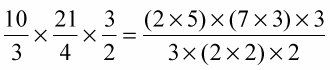3. Cross out any common factors.

In this example, you cross out 2 and 3 because they’re common factors — that is, they appear in both the numerator and denominator: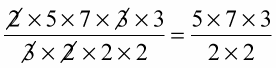4. Multiply the numerators together and the denominators together.